# PANDAS 数据合并与重塑（concat篇）数据分析 同时被 3 个专栏收录2 篇文章 3 订阅

pandas作者Wes McKinney 在【PYTHON FOR DATA ANALYSIS】中对pandas的方方面面都有了一个权威简明的入门级的介绍，但在实际使用过程中，我发现书中的内容还只是冰山一角。谈到pandas数据的行更新、表合并等操作，一般用到的方法有concat、join、merge。但这三种方法对于很多新手来说，都不太好分清使用的场合与用途。今天就pandas官网中关于数据合并和重述的章节做个使用方法的总结。

• 文中代码块主要有pandas官网教程提供。

# 1 concat

concat函数是在pandas底下的方法，可以将数据根据不同的轴作简单的融合

pd.concat(objs, axis=0, join='outer', join_axes=None, ignore_index=False,
keys=None, levels=None, names=None, verify_integrity=False)

objs: series，dataframe或者是panel构成的序列lsit
axis： 需要合并链接的轴，0是行，1是列
join：连接的方式 inner，或者outer

## 1.1 相同字段的表首尾相接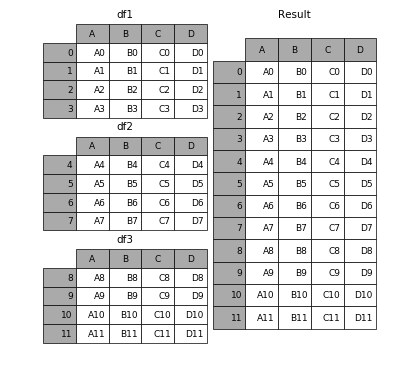# 现将表构成list，然后在作为concat的输入
In : frames = [df1, df2, df3]

In : result = pd.concat(frames)

In : result = pd.concat(frames, keys=['x', 'y', 'z'])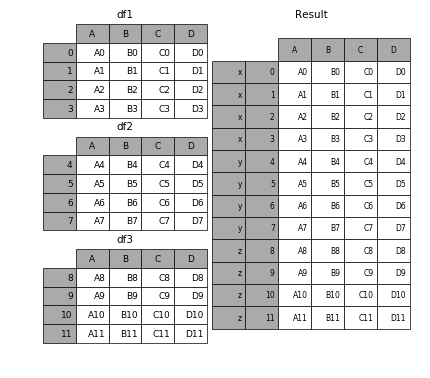## 1.2 横向表拼接（行对齐）

### 1.2.1 axis

In : result = pd.concat([df1, df4], axis=1)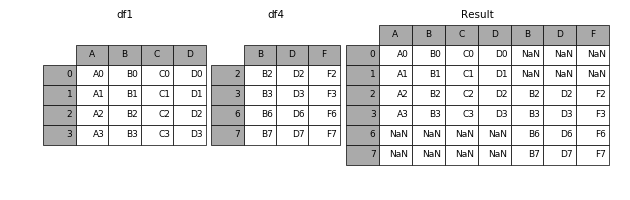### 1.2.2 join

In : result = pd.concat([df1, df4], axis=1, join='inner')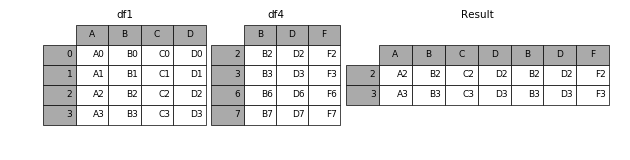### 1.2.3 join_axes

In : result = pd.concat([df1, df4], axis=1, join_axes=[df1.index])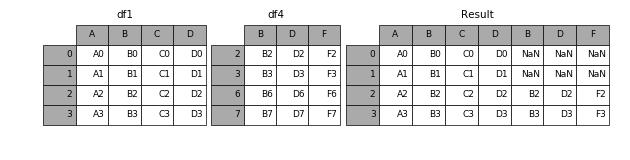## 1.3 append

append是series和dataframe的方法，使用它就是默认沿着列进行凭借（axis = 0，列对齐）

In : result = df1.append(df2)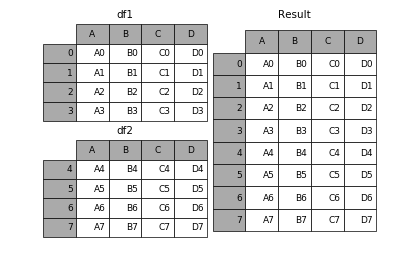## 1.4 无视index的concat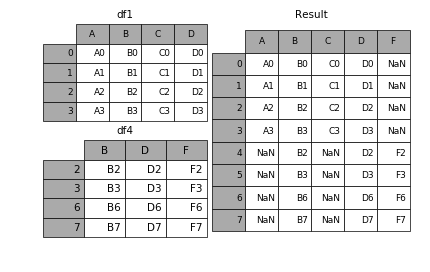## 1.5 合并的同时增加区分数据组的键

### 1.5.1 可以直接用key参数实现

In : result = pd.concat(frames, keys=['x', 'y', 'z'])### 1.5.2 传入字典来增加分组键

In : pieces = {'x': df1, 'y': df2, 'z': df3}

In : result = pd.concat(pieces)## 1.6 在dataframe中加入新的行

append方法可以将 series 和 字典就够的数据作为dataframe的新一行插入。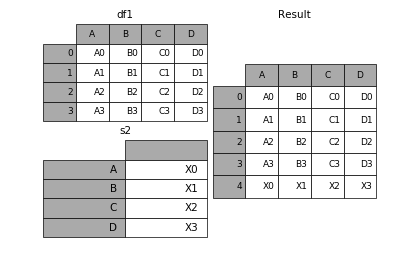In : s2 = pd.Series(['X0', 'X1', 'X2', 'X3'], index=['A', 'B', 'C', 'D'])

In : result = df1.append(s2, ignore_index=True)

## 表格列字段不同的表合并

如果遇到两张表的列字段本来就不一样，但又想将两个表合并，其中无效的值用nan来表示。那么可以使用ignore_index来实现。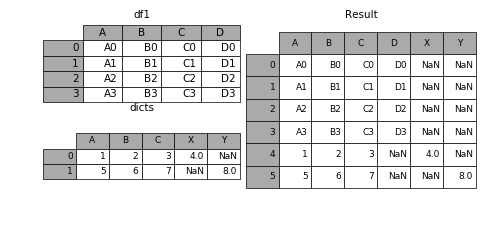In : dicts = [{'A': 1, 'B': 2, 'C': 3, 'X': 4},
....:          {'A': 5, 'B': 6, 'C': 7, 'Y': 8}]
....:

In : result = df1.append(dicts, ignore_index=True)03-173万+
02-0113万+
01-171万+
09-178195
06-063万+
05-231万+
01-15969
05-201万+
07-207917
01-111924
11-01531
02-231873
10-105502
06-20155
09-222281
09-061340点击重新获取扫码支付余额充值